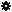﻿ Object Model: PolygonFace.NeighborVertices

PolygonFace.NeighborVertices

## PolygonFace.NeighborVerticesv3.0

### Description

Returns a collection of Vertex neighbors within a given distance. If the distance is 1 all Vertex objects comprising the current PolygonFace are returned in counter-clockwise order.

### C# Syntax

 ```VertexCollection PolygonFace.NeighborVertices( Int32 in_lDistance ); ```

### Scripting Syntax

 ```oReturn = PolygonFace.NeighborVertices( [Distance] ); ```

### Parameters

Parameter Type Description
Distance Integer Distance is an integer value representing the degree of neighborhood (eg: degree=2 for a polygon means its adjacent vertices plus the adjacent vertices of the adjacent polygons)

Default Value: 1

### Examples

#### VBScript Example

 ```set oCube = ActiveSceneRoot.AddGeometry( "Cube", "MeshSurface" ) set oGeometry = oCube.ActivePrimitive.Geometry for each Polygon in oGeometry.Polygons set oNeighbors = Polygon.NeighborVertices( 1 ) str = "Vertices neighbors of face( " & Polygon.index & ") are :" for each n in oNeighbors str = str & " " & n.index next logmessage str next ```